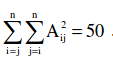# GATE | GATE-CS-2017 (Set 1) | Question 45

• Last Updated : 17 Aug, 2021

Let A be m×n real valued square symmetric matrix of rank 2 with expression given below.Consider the following statements


(i)  One eigenvalue must be in [-5, 5].
(ii) The eigenvalue with the largest magnitude
must be strictly greater than 5.


Which of the above statements about engenvalues of A is/are necessarily CORRECT?
(A) Both (i) and (ii)
(B) (i) only
(C) (ii) only
(D) Neither (i) nor (ii)

Explanation: As a rank of A matrix = 2, hence

=> n-2 eigen values are zero. Letbe the eigen values.
Given that————(1)

We know thatTrace of (AA)T = Trace of A2 (since A is symmetric) =————(2)

From (1) and (2) :Hence, atleast one of the given eigen values lies in [-5,5](only 1 is correct).

This solution is contributed by Sumouli Chaudhary.

Quiz of this Question

My Personal Notes arrow_drop_up
Recommended Articles
Page :